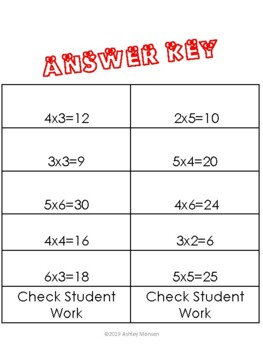# Grouping Multiplication Strategy PracticeSubject
Resource Type
File Type

PDF

(743 KB)
Product Rating
Standards
Also included in:
1. This bundle includes whole group multiplication anchor charts that can be used all year long in your classroom. Pages with the individual strategies are meant to be hung in your classroom for a whole group experience and used to teach the strategies and anchor students all year long. The first pag
\$12.50
\$10.50
Save \$2.00
• Product Description
• StandardsNEW

This is a practice sheet for the grouping multiplication strategy. It can be used as a whole group practice or as a practice in a math center. Answer key is included.

Identify arithmetic patterns (including patterns in the addition table or multiplication table), and explain them using properties of operations. For example, observe that 4 times a number is always even, and explain why 4 times a number can be decomposed into two equal addends.
Fluently multiply and divide within 100, using strategies such as the relationship between multiplication and division (e.g., knowing that 8 × 5 = 40, one knows 40 ÷ 5 = 8) or properties of operations. By the end of Grade 3, know from memory all products of two one-digit numbers.
Determine the unknown whole number in a multiplication or division equation relating three whole numbers. For example, determine the unknown number that makes the equation true in each of the equations 8 × ? = 48, 5 = __ ÷ 3, 6 × 6 = ?.
Use multiplication and division within 100 to solve word problems in situations involving equal groups, arrays, and measurement quantities, e.g., by using drawings and equations with a symbol for the unknown number to represent the problem.
Interpret products of whole numbers, e.g., interpret 5 × 7 as the total number of objects in 5 groups of 7 objects each. For example, describe a context in which a total number of objects can be expressed as 5 × 7.
Total Pages
N/A
N/A
Teaching Duration
N/A
Report this Resource to TpT
Reported resources will be reviewed by our team. Report this resource to let us know if this resource violates TpT’s content guidelines.